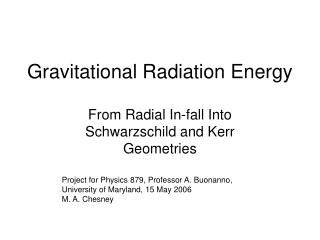DownloadDownload PresentationGravitational Radiation Energy

# Gravitational Radiation Energy

Download Presentation## Gravitational Radiation Energy

- - - - - - - - - - - - - - - - - - - - - - - - - - - E N D - - - - - - - - - - - - - - - - - - - - - - - - - - -
##### Presentation Transcript

1. Gravitational Radiation Energy From Radial In-fall Into Schwarzschild and Kerr Geometries Project for Physics 879, Professor A. Buonanno, University of Maryland, 15 May 2006 M. A. Chesney

2. Motivation • Radial in-fall simplifies calculations tremendously • Total energy output and waveforms calculable by multiple methods • Results of various methods and authors easy to compare • Intense source of gravitational radiation • May be first detected by LIGO • Reaches limits of mass-energy to GW conversion • Look at size and shape of craters on the moon!

3. Newton • No GW, action at a distance, instantaneous propagation • But, as derived in class combine with Linearized Gravity gives fairly good result! Solving the differential equation, setting Rs = 2 G M / c2, and z[tmax] = R

4. Linearized Gravity Given the energy output and the Mass Quadrupole Moment We integrate from -infinity to tmax and get Setting R = Rs the prediction is 0.019 m c2 (m / M)

5. Mathematica code

6. Mathematica code, cont.

7. Mathematica code, cont. The above result is about twice the value predicted when the background curved spacetime is taken into account. See Animation for a hint as to why***

8. Radial In-fall into a Schwarzschild Black Hole Using Curved Background • Davis, Ruffini, Press and Price  were among the first to accurately describe the radial in-fall problem in terms of the wave form of the gravitational radiation and the total energy of the outgoing waves. • Employed Fourier transformations of metric perturbations crafted by Regge and Wheeler. • Interprets the gravity wave generation as the result of vibrations or quasinormal modes in the horizon caused by the impacting mass. • Paper did not detail the methods for solution or give the explicit amplitude solutions for any of the modes but they did give an overall result of • Etotal = 0.0104 m c2 (m / M) . • Listed methods as direct integration of Zerilli wave equation with a numerical search technique so that incoming waves only at Rs and outgoing waves only at infinity are found. Also Greens function technique. • Next slide shows equations to be solved.

9. Analytic perturbation mathematical methods

10. Relativistic In-fall to Schwarzschild • Smarr  predicted a zero-frequency limit (ZFL) dE/dw, w -> 0 that is easily calculable for masses with a non-zero velocity at infinity • Able to make an order of magnitude estimate of energy for each polarization and the angular dependence. • Cardoso confirms ZFL of Smarr, and estimate of energy using Regge-Wheeler-Sasaki formalism • (dE/dw) w -> 0 = 0.4244m2 c2g2 • Etotal = 0.26m c2g2 (m / M) • Perturbation calculation gives excellent agreement with Smarr’s ZFL method as v -> 1 at infinity. • (dE/dw) w -> 0 = 4/(3 p) m2 c2g2 = 0.4244m2 c2g2 • Etotal = 0.2m c2g2 (m / M) • Next few slides detail Smarr’s ZFL method.

11. Infall Along the Z-Axis of a Kerr Black Hole • Nakamura and Sasaki  have taken the analysis a step further and found the gravitational radiation produced by the in-fall of a non-rotating test mass along the z-axis of a spinning black hole. • Using a finite difference method they found the total energy radiated • 0.0170m c2 (m / M) when a = 0.99. • Cardoso and Lemos  used the above Sasaki-Nakamura formalism to find the gravitational radiation when the impacting object is highly relativistic at infinity. • They found that for a collision along the symmetry axis the total energy is 0.31m c2g2 (m / M) for a = 0.999 M. • The equatorial limit is 0.69m c2g2 (m / M) for a = 0.999 M, v -> 1 • Represents the most efficient gravitational wave generator in the universe • See animation for hint why Equatorial > Radial GW generator****

12. GW From spinning particle into spinning BH • Mino, Shibata, Takahiro  examined z-axis, 0 in-fall velocity case • 0.0106m c2(m / M) , Parallel a = 0.99 M and m • 0.0298m c2(m / M) , Antiparallel a = 0.99 M and m • The parallel spin case is nearly same as spin zero case of Davis in 1971 • 0.0104 m c2 (m / M) • Intermediate to result when no spin falls into spin = 0.99 M • 0.0170m c2 (m / M) • Two factors at work here • Spin-spin interaction described by Papapetrou-Dixon equations • Energy-momentum tensor of the spinning particle • Magnificent treatment, combining Papapetrou-Dixon equations with Teukolski formalism, using the Sasaki-Nakamura formulation